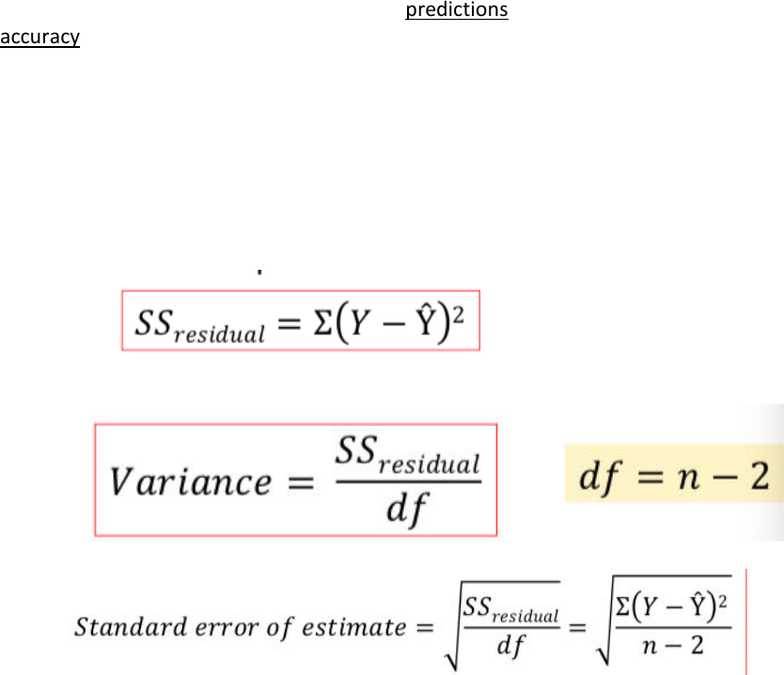Study Guides (390,000)
CA (150,000)
UTSG (10,000)
PSY (800)
Amanda (10)
Final

# PSY202H1 Study Guide - Final Guide: Linear Regression, Squared Deviations From The Mean, Pearson Product-Moment Correlation Coefficient

Department
Psychology
Course Code
PSY202H1
Professor
Amanda
Study Guide
Final

This preview shows half of the first page. to view the full 3 pages of the document.Page 26:
What is the name of beta?
Standardized regression coefficient.
Beta is equal to the Pearson correlation r.
Why the best fitting line is not always a good-fitting line?
Because how accurate the prediction is depending on how well the points on the regression line
corresponding to the real data. The regression line that we calculated are the least squared
error, but this still depends on the natural of the point. If the points are originally close and
trended like a line, you give a regression line much like it, then it will be a good fitting line
compares to a graph that has dots all over.
Regression & prediction:
Regression equation only allows us to make predictions but does not tell us anything about the
accuracy of those predictions.
How to measure the accuracy of the regression?
We will compute the standardized error of estimate. The standard error of estimate is a
measure of the standard distance between the predicted Y-values on the regression line and
the actual Y-values in the set of data.
The calculation of the standard error of estimate& how it is similar to the standard deviation:
1. find the sum of squared deviations (SS)
2. Variance (SS/df)
3. Take the square root of the variance
Why the df = n-2?
Because there are 2 things being estimated (intercept and the slope) in order to obtain the sum
of squares.